# How to Perform a Repeatability Test for Estimating Uncertainty in Measurement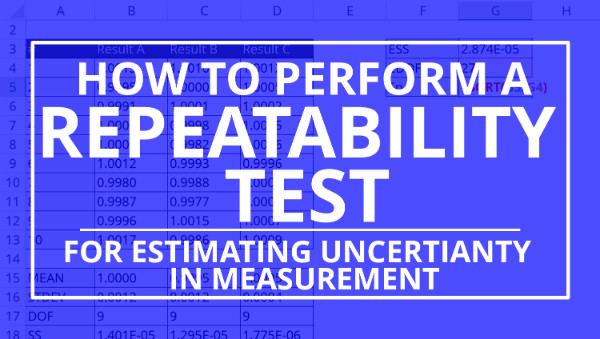## INTRODUCTION

Performing a repeatability test is an essential part of estimating uncertainty in measurement. It is the most common experiment performed to collect Type A Uncertainty data.

Additionally, most accreditation bodies require that you include repeatability data in your uncertainty budgets. However, many people have trouble with performing a repeatability test. They do not know how to conduct the experiment, collect the data, or analyze the results.

In this guide, you are going to learn everything that you need to know about repeatability testing;

1. What is a repeatability test,
2. How to perform a repeatability test, and
3. How to calculate repeatability.

Plus, I have created some great tools to help you perform a repeatability test and analyze the results next time you need to estimate measurement uncertainty.

## BACKGROUND

Every day, I work with clients who need my help to estimate uncertainty in measurement. During the process, I typically ask clients to perform repeatability and reproducibility testing.

However, many of my clients do not know how to conduct a repeatability test. So, they ask for procedures, checklists, and consultation.

After years of continually helping clients perform a repeatability test, I noticed that I had not written a formal guide to help these clients; nor automate the process.

Therefore, I decided to create a guide dedicated to repeatability testing in order to answer all of the questions that I have been asked.

In this guide you will learn;

## WHAT IS TYPE A UNCERTAINTY

Type A Uncertainty is a component of uncertainty where data is collected from a series of observations and evaluated using statistical methods associated with the analysis of variance (ANOVA).

Commonly referred to as Type A Data, Type A Uncertainty is typically associated with the results of Repeatability and Reproducibility Testing. However, it can also be associated with stability testing.

According to the Vocabulary in International Metrology (VIM), Type A Evaluation of measurement uncertainty is a component of measurement uncertainty evaluated by a statistical analysis of measured quantity values that were obtained under defined measurement conditions.## WHAT IS A REPEATABILITY TEST

A repeatability test is an experiment performed to evaluate how repeatable your results are under a set of similar conditions.

When performing a repeatability test, you will want to collect data using the;

1. Same method,
2. Same operator,
3. Same equipment,
4. Same environmental conditions,
5. Same location, and
6. Same item or unit under test.

Essentially, you want to collect repeatable results over a short period of time without changing anything (if possible).

According to the Vocabulary in International Metrology (VIM), measurement repeatability is measurement precision under a set of repeatable conditions of measurement.Furthermore, the VIM defines a repeatability condition of measurement as a condition of measurement, out of a set of conditions that includes the same measurement procedure, same operators, same measuring system, same operating conditions, same location, and same replicate measurement on the same or similar objects over a short period of time.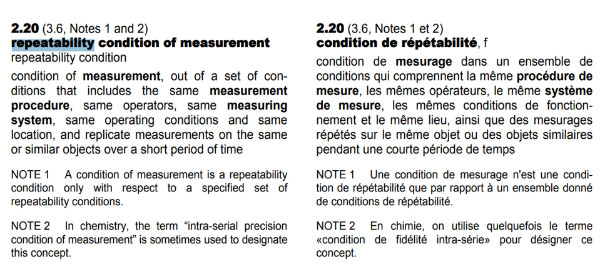Therefore, you need to define your measurement conditions and collect repeatable results over a short period time so you can evaluate the precision of your process.

In the next section, you will learn step by step how to perform a repeatability test.

## HOW TO PERFORM A REPEATABILITY TEST

To calculate repeatability, you need to have a procedure.

Similar to every test or measurement performed in your laboratory, you must have a method or procedure to guide you through the process and ensure consistency in your results.

In this section, you will learn to perform a repeatability test step by step. Follow the instructions below to add repeatability test data to your uncertainty budgets.

Here is a list of the steps in this process;

1. Select the measurement function to test,
2. Select the measurement range,
3. Select the test-point(s),
4. Select the method,
5. Select the equipment,
6. Select the operator,
7. Perform the test,
8. Collect the number n of repeated samples,
10. Save a record of your results (recommended),

### 1. Select the Measurement Function

Before you begin performing a repeatability test, it is a good idea to determine what you are going to test.

Start by selecting the measurement function that will be tested.

The measurement function will be the category that best describes your measurement or test result, such as;

1. DC Voltage Generate/Measure
2. Length
3. Pressure Generate/Measure
4. Torque Generate/Measure
5. Temperature Source/Measure

If you are having trouble, take a look at your scope of accreditation (or another laboratory’s scope of accreditation) and pick the measurement function that you would like to test.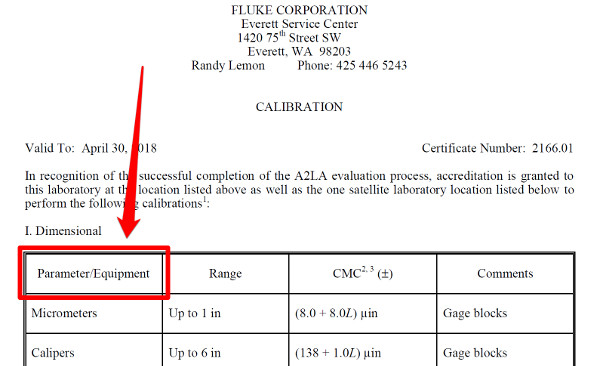### 2. Select the Measurement Range

After selecting the measurement function, pick a measurement range to test. This should consist of a chosen starting measurement value and an ending measurement value; typically low to high.

I recommend that you pick a measurement range listed in your scope of accreditation or in the equipment manufacturer’s specifications.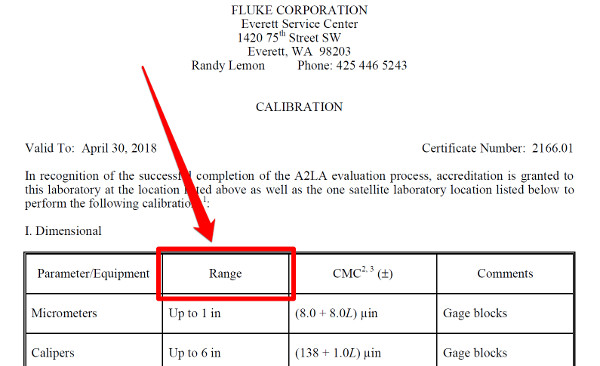### 3. Select the Test Points

Now that you have specified a measurement range, it is time to select the test-points for your repeatability test.

If you assume that your measurement function is linear, you will need to select two test-points along the measurement range. Typically, it should be a low value and a high value.

Some common practices are to select test points that are at 10% and 90% of the measurement range or at 20% and 100% of the measurement range. For best results, I recommend that you select two calibration points along the measurement range (if available).

If you assume that your measurement function is non-linear, you may want to select three of more test points to evaluate. This will help you prevent errors due to the curvature of the measurement function.

Should you decide to perform a repeatability test at three of more test points, try to select evenly spaced test points to prevent modeling errors in your CMC Uncertainty prediction equation.

### 4. Select the Method

The next step to performing a repeatability test is to select the measurement method or procedure. You will want to select a method or procedure that best represents the how the measurement process is performed.

A good place to start is to use a test method or calibration procedure that best represents your measurement process. This will help you make sure that you are evaluating a measurement process that you would normally perform in your laboratory.

If you do not have a procedure, try writing one for your process. Even if the procedure minimally covers the steps of the process, it is still better than nothing. Additionally, it will help you get consistent results.

### 5. Select the Equipment

Following the chosen method, select the equipment recommended to perform your measurement process. Make sure that your equipment is calibrated and functioning properly before use.

For best results, select the most accurate equipment available to you. The equipment you choose will affect the results. So, select your best equipment.

### 6. Select the Operator

Select an operator to perform the repeatability test. Pick an operator that is qualified and experienced at performing the test or measurement.

Typically, your most experienced or qualified operators will yield the best results.

Your goal should be to achieve consistent repeatable results. Therefore, choose an operator that you will help you achieve it.

### 7. Perform the Repeatability Test

Now that you have established all of your conditions, it is time to perform a repeatability test for the measurement function, range, and test-points that you selected using the method, equipment, and operator that you selected.

Perform steps eight through eleven to conduct a repeatability test.

### 8. Collect ‘n’ Number of Samples and Record Your Results

When performing a repeatability test, collect a defined number of repeated samples. Typically, it is recommended that you collect at least 20 to 30 samples to obtain statistically significant results.

However, collecting 20 to 30 samples is not always practical for every test or measurement. Instead, collect the number of samples that is most appropriate for your situation and measurement system.

If you can only collect 5 samples because the test or measurement process is time-consuming or labor-intensive, then only collect 5 samples. If you can collect 100 samples because your test or measurement process is quick and automated, then collect 100 samples.

Only collect the number of samples that is most appropriate for your situation.

After collecting samples, you will need to analyze your data using the analysis of variance (ANOVA). Calculate the mean (i.e. average), standard deviation, and the degrees of freedom.

If you are analyzing a single set of data, you will use the calculated standard deviation and degrees of freedom in your uncertainty budget.

If you are analyzing multiple sets of data, you will need to use the method of pooled variance to calculate the pooled standard deviation and degrees of freedom for your uncertainty budget.

### 10. Save a Record of Your Results (Recommended)

Anytime that you collect data, it is a good idea to save a record of your results. It will come in handy if you need to go back and review your results or compare them with other repeatability test data.

I always recommend that you keep files for your repeatability test data. However, you do not have to keep records. Instead, you can just collect Type A data each time you update your uncertainty budgets. The choice is yours.

Finally, add your repeatability test results to your uncertainty budgets. Create a line item for repeatability and include the standard deviation and the degrees of freedom in your budget.

Characterize your repeatability results with a Normal distribution where ‘k’ equals one (i.e. k=1).

For step by step instructions, read the section below: How to Add Repeatability to Your Uncertainty Budget.

## HOW MANY SAMPLES SHOULD YOU COLLECT

A common question people ask when performing a repeatability test is, “How many samples should I collect?”

The answer is, “As many as you practically can.”

As a general rule of thumb, it is typically recommended to collect 20 to 30 samples to be statistically sound. However, this rule is not applicable to every scenario.

If you are using automation and have the capability to collect 100 or more samples over a short period of time, then collect 100 or more samples. It is practical for your test or measurement process.

If you are performing a test or measurement that is difficult or time-consuming, it may be hard to collect 20 to 30 samples. Therefore, you should collect fewer samples. In this situation, it may be more practical to only collect three to five samples.

Make sure to select the number of samples that is appropriate for your measurement process.

If you would like to manipulate your results to achieve a desired margin of error (i.e. standard deviation), use the formula below;How to Calculate
1. Choose your desired confidence level (z).
2. Choose your desired margin of error (MOE).
3. Multiply the result of step 1 by the value by the standard deviation of the sample set.
4. Divide the result by the margin of error selected in step 2.
5. Square the result calculated in step 4.

## HOW TO COLLECT REPEATED SAMPLES

When performing a repeatability test, some people get confused on how to collect repeated samples. They believe that they must;

1. Set-up a test,
2. Collect a result,
3. Break-down the setup, and
4. Repeat the process ‘n’ number of times.

This is not true.

Conducting a repeatability test following that process would be rigorous and time-consuming.

Instead, think about how you could collect the data easier and faster if you were to follow this process;

1. Set-up a test,
2. Collect a result,
3. Repeat ‘n’ number of times, and
4. Break-down the setup.

If you follow this process, you would be able to complete a repeatability test much faster. So, to make repeatability testing less rigorous, make sure to collect repeated samples back to back over a short period of time.

If your measurement equipment repeatedly samples results and refreshes the display, collect ‘n’ number of displayed results back to back over a short period of time.

Should your measurement equipment require manual sampling, repeat the process over and over again until you collect your desired number of samples.

Do not break down your test set-up each sample and repeat. That process would actually be a form of reproducibility testing, not repeatability testing.

## HOW TO CALCULATE REPEATABILITY (SINGLE TEST)

Analyzing the results of a single repeatability test is pretty simple. Just calculate the mean, standard deviation, degrees of freedom.

1. Calculate the Mean,
2. Calculate the Standard Deviation,
3. Calculate the Degrees of Freedom.

In the sections below, you will learn how to perform these calculations in Excel.

### 1. Calculate the Mean using ExcelHow to Calculate
1. Select a cell to calculate the mean.
2. Type “=AVERAGE(“ in the cell.
3. Select the cells containing your results.
4. Type “)“ and press the “Enter” key.

### 2. Calculate the Standard Deviation using ExcelHow to Calculate
1. Select a cell to calculate the standard deviation.
2. Type “=STDEV(“ in the cell.
3. Select the cells containing your results.
4. Type “)“ and press the “Enter” key.

### 3. Calculate the Degrees of Freedom using ExcelHow to Calculate
1. Select a cell to calculate the degrees of freedom.
2. Type “=COUNT(“ in the cell.
3. Select the cells containing your results.
4. Type “)-1“ and press the “Enter” key.

## HOW TO CALCULATE REPEATABILITY (MULTIPLE TESTS)

Analyzing the results of a several repeatability tests is more complex. You cannot average standard deviation, so you must pool them together using the method of pooled variance.

1. Calculate the Mean,
2. Calculate the Standard Deviation,
3. Calculate the Degrees of Freedom,
4. Calculate the Pooled Standard Deviation.

### 1. Calculate the Mean using ExcelHow to Calculate
1. Select a cell to calculate the mean.
2. Type “=AVERAGE(“ in the cell.
3. Select the cells containing your results.
4. Type “)“ and press the “Enter” key.

### 2. Calculate the Standard Deviation using ExcelHow to Calculate
1. Select a cell to calculate the standard deviation.
2. Type “=STDEV(“ in the cell.
3. Select the cells containing your results.
4. Type “)“ and press the “Enter” key.

### 3. Calculate the Degrees of Freedom using ExcelHow to Calculate
1. Select a cell to calculate the degrees of freedom.
2. Type “=COUNT(“ in the cell.
3. Select the cells containing your results.
4. Type “)-1“ and press the “Enter” key.

### 4. Calculate the Pooled Standard Deviation Using Excel

#### 4a. Calculate the Weighted VarianceHow to Calculate
1. Select a cell to calculate the weighted variance (i.e. SS).
2. Type “=CELL1*CELL2^2“ in the cell.
3. Copy and Paste function into other cells.

#### 4b. Calculate the Total Weighted VarianceHow to Calculate
1. Select a cell to calculate the total weighted variance.
2. Type “=SUM( “ in the cell.
3. Select the cells containing your results.
4. Type “)“ and press the “Enter” key.

#### 4c. Calculate the Total Degrees of Freedom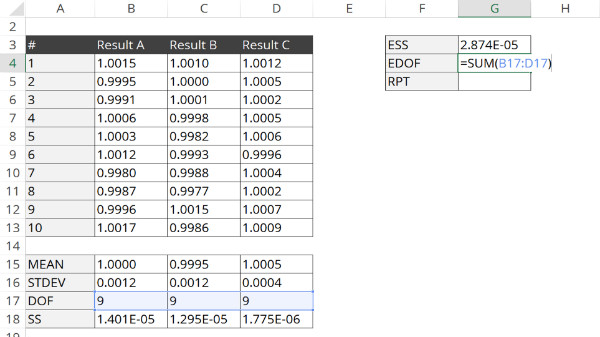How to Calculate
1. Select a cell to calculate the total degrees of freedom.
2. Type “=SUM( “ in the cell.
3. Select the cells containing your results.
4. Type “)“ and press the “Enter” key.

#### 4d. Calculate the Pooled Standard Deviation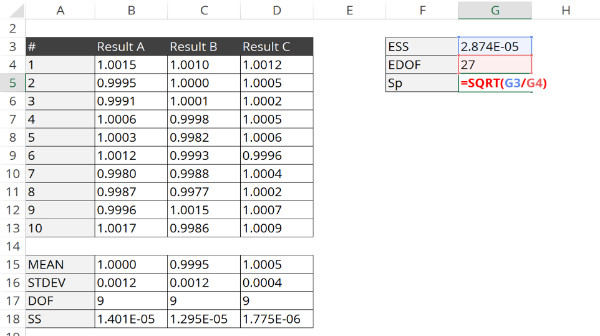How to Calculate
1. Select a cell to calculate the pooled standard deviation.
2. Type “=SQRT( “ in the cell.
3. Select the cell containing the total weighted variance (i.e. ESS).
4. Type “/ “ for the divide function.
5. Select the cell containing the total degrees of freedom (i.e. EDOF).
6. Type “)“ and press the “Enter” key.

The pooled standard deviation will be your value for repeatability, and the total degrees of freedom will be your value for degrees of freedom.

After performing a repeatability test and analyzing your data, you will want to add the results to your uncertainty budget.

To complete this task, just follow the step by step instructions listed below. The instructions should work for any ISOBudgets uncertainty budget calculators and most uncertainty budget calculators offered by other organizations.

If you don’t have an uncertainty budget calculator, you can buy one here.

### 1. Add a line item to your uncertainty budget for repeatability,### 2. Enter your sensitivity coefficient for repeatability (typically “1”),### 3. Enter your uncertainty value (i.e. standard deviation) to the uncertainty budget,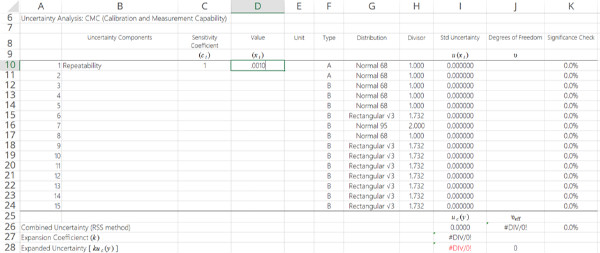### 4. Enter the unit of measure,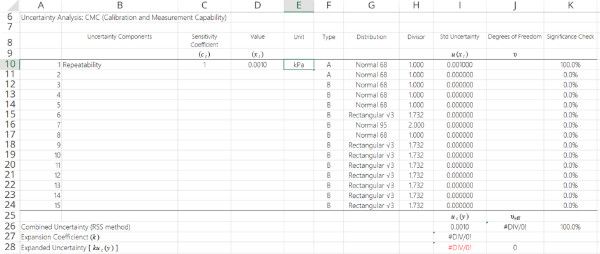### 5. Enter the Uncertainty Type (typically “A”),### 6. Select the “Normal“ probability distribution,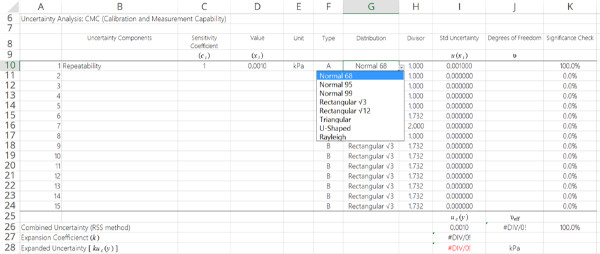### 7. Select the divisor where k=1,### 8. Enter your degrees of freedom.## REPEATABILITY TEST CHECKLIST & CALCULATOR

Are you ready perform a repeatability test?

If so, get the repeatability test checklist and calculator. The checklist will give a summary of all the instructions provided in this guide, and the calculator will help you analyze your results so you can calculate repeatability faster.

## CONCLUSION

Performing a repeatability test is the most common methods used to collect type A uncertainty data. If your laboratory is ISO/IEC 17025 accredited or plans to get accredited, you will need to perform repeatability testing and include the results in your uncertainty budgets.

In this guide, you should have learned how to perform a repeatability test and analyze the results to calculate measurement repeatability. Plus, you should have learned some additional tips to help you complete the process with more confidence.

Calculating repeatability is not hard, but it is a task that some people find difficult to complete. Use the information and tools provided in this guide to help you estimate uncertainty in measurement faster and get prepared for accreditation.

Posted in: